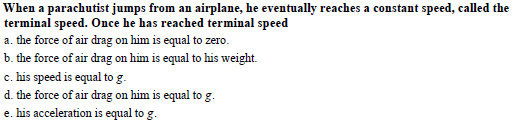When a parachutist jumps from an airplane, he eventually reaches a constant speed, called theterminal speed. Once he has reached terminal speeda. the force of air drag on him is equal to zero.b. the force of air drag on him is equal to his weight.c. his speed is equal to gd. the force of air drag on him is equal to ge. his acceleration is equal to g

Questionhelp_outlineImage TranscriptioncloseWhen a parachutist jumps from an airplane, he eventually reaches a constant speed, called the terminal speed. Once he has reached terminal speed a. the force of air drag on him is equal to zero. b. the force of air drag on him is equal to his weight. c. his speed is equal to g d. the force of air drag on him is equal to g e. his acceleration is equal to g fullscreen
Step 1

As a parachute jumps from an airplane, his velocity is increasing. This time, there will be acceleration. Then it attains a constant speed. From this moment onwards, there will be no acceleration. After attaining constant speed, since there is no net force, parachutist is said to be in equilibrium.

Step 2

The force on air drag cannot be zero. If it is zero, parachutist will definitely accelerated and there will be non-zero net force acting on it. Thus, option (a) is incorrect.

There are two forces acting on the parachutist. The weight of parachutist acting vertically downwards and air drag acting vertically upwards. At equilibrium condition, it will balance each other. Thus, option (b) is correct.

Speed and gravitational acceleration (g) ...

Want to see the full answer?

See Solution

Want to see this answer and more?

Our solutions are written by experts, many with advanced degrees, and available 24/7

See Solution
Tagged in

Physics